# A math problem that apparently a 13 year old can solve and I can't, lol...

Status
Not open for further replies.

#### Aurelia

##### I'm. Not. Like. You. I. Just. Fuck. Up.
Okay, so I already know the answer to this because it was given, but I was trying to figure out HoW to actually solve it and show my work in the process, and after about an hour of struggling, I couldn't freaking do it. Needless to say, I feel like a total dumbass. This is an algebra problem given to a 13 year old from Russia, so I blame America's school system (that's my excuse and I'm sticking to it ;P)

Okay, so this is the math problem:
@Auri I know you'll probably solve it in like 10 seconds and make me feel like even more of a dumbass, haha.

But okay, so there are 3 plates in total with apples on them. The first plate has 1/2 of the total number of apples on all 3 plates. If you take away 1/2 of the number of apples that lie on the second plate from the first plate, as well as 1/2 of the number of apples that lie on the third plate also from the first plate, then only 2 apples would be left over on the first plate. How many apples are on each plate?

Like i said, I already know the answer, i just want to see how someone solves the problem using algebra (let's call the plates X, Y, and Z -- how would you solve for X, Y, and Z with the information given in the problem?) I figured out fow to solve for X, but then after that, my dumb ass seems to get confused. So would someone who is better than me at math please show me how they would do this because it's pissing me the hell off that a 13 year old can do algebra better than I can :P Shit...when I was 13, we weren't doing algebra problems this difficult yet. Goes to show why basically all other countries are smarter than us, eh?

#### Auri

##### 🎸🎼Metal Star🎼🎸
Safety & Support
SF Supporter
Well you tell me the exact answer.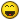Cause it seems like an indeterminate system (more than one solution) with too many unknowns given the information. We don't know anything about y and z.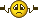So if I understood correctly, it should get to all solutions verifying x = 4 and y + z = 4. Assuming we only have whole apples (so removing fractions, lol), it works for :

x = 4, y = 0, z = 4
x = 4, y = 1, z = 3
x = 4, y = 2, z = 2
x = 4, y = 3, z = 1
x = 4, y = 4, z = 0

I may be misunderstanding though, or there is a magic trick I'm missing, but that's what I have at first glance.

Yeah that kind of thing is taught around 13-14, but it doesn't make you dumb not to know how to solve it as an adult, we forget most of what we learn in school.

#### Pebble mouse

##### ¯\_(ツ)_/¯
we forget most of what we learn in school.
OMG, it's good to know I'm not alone!! I can barely remember a thing from school or my diploma for that matter!

#### Auri

##### 🎸🎼Metal Star🎼🎸
Safety & Support
SF Supporter
OMG, it's good to know I'm not alone!! I can barely remember a thing from school or my diploma for that matter!
You're not alone at all, it's everyone's case. If someone remembers something, they've been using it directly or indirectly on a regular basis since then. Nothing to be ashamed of.#### ameliaQ

SF Supporter
I found only one possible solution

#### Attachments

• 1.8 MB Views: 15
• 1.8 MB Views: 15
• 1.7 MB Views: 13

#### Witty⭐️Sarcasm ⭐️

SF Supporter
You're not a dumbass, I literally don't understand algebra at all. Trying to figure out those equations makes my head hurt, lol.

#### Auri

##### 🎸🎼Metal Star🎼🎸
Safety & Support
SF Supporter
I found only one possible solution
Yeah I agree with all equations.Except that the true statement at the end confirms that x = 4, but not necessarily that y = z (why would they have to be equal?). All we know is that they have to satisfy y + z = 4. Or?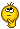#### ameliaQ

SF Supporter
so, going back to the equation y+z=4, we rearranged it to isolate one variable (z). then because it is a system of equations, we can substitute the new z value of 4-y into the initial equation of y+z=4 to simplify the equation from 2 variables to 1 variable. we put the variable z in terms of x. then when we solved the simplified equation that only has 1 variable, we found the solution to be a statement that is always true (4=4 in this case). what this technically means in our example is that the value of apples on plate y will always be the same as on plate z. but since the system is not only based on 2 plates, but 3, we have to bring back consideration for plate x which we already found had to contain 4 apples. the initial information in the question told us that the amount of apples on plate x (4) has to equal the total of apples on plates y and z combined (y+z=4). and since we found that y and z always have to be the same value (because 4=4), we can also say that y+y=4 and z+z=4, which means that y=2 and z=2. hope that makes sense.

#### Auri

##### 🎸🎼Metal Star🎼🎸
Safety & Support
SF Supporter
what this technically means in our example is that the value of apples on plate y will always be the same as on plate z.
Okay, instead of contradicting you... Can I just ask why that is? About that technical part?#### Livelife

SF Supporter
Okay, so I already know the answer to this because it was given, but I was trying to figure out HoW to actually solve it and show my work in the process, and after about an hour of struggling, I couldn't freaking do it. Needless to say, I feel like a total dumbass. This is an algebra problem given to a 13 year old from Russia, so I blame America's school system (that's my excuse and I'm sticking to it ;P)

Okay, so this is the math problem:
@Auri I know you'll probably solve it in like 10 seconds and make me feel like even more of a dumbass, haha.

But okay, so there are 3 plates in total with apples on them. The first plate has 1/2 of the total number of apples on all 3 plates. If you take away 1/2 of the number of apples that lie on the second plate from the first plate, as well as 1/2 of the number of apples that lie on the third plate also from the first plate, then only 2 apples would be left over on the first plate. How many apples are on each plate?

Like i said, I already know the answer, i just want to see how someone solves the problem using algebra (let's call the plates X, Y, and Z -- how would you solve for X, Y, and Z with the information given in the problem?) I figured out fow to solve for X, but then after that, my dumb ass seems to get confused. So would someone who is better than me at math please show me how they would do this because it's pissing me the hell off that a 13 year old can do algebra better than I can :P Shit...when I was 13, we weren't doing algebra problems this difficult yet. Goes to show why basically all other countries are smarter than us, eh?
I thought I had the answer with 8/4/4 since plate 2 and 3 have to start out with being even numbers so I then plugged in 4/2/2.. it seems to work. No formula, just plugging in. I made 3 attempts.

#### Auri

##### 🎸🎼Metal Star🎼🎸
Safety & Support
SF Supporter
Okay, that is not what this is, 13 year-olds certainly don't do that... Here they try to solve for parameters a, b, c... when the variable x is indeterminate, there is still an infinite amount of solutions for x (like the definition of an identity states).

It's a different thing than solving a system with 3 variables once you've determined the solution for one of them (x = 4) but still can't determine the two others.

All you can conclude is that y + z = 4. There is no reason to conclude that y and z have the exact same value just because they've disappeared from the equation and you get to an identity that is always true. It actually means you can't determine their values. (unless you can explain that rule, I'm open to being proven wrong ^^)

If you try for values 4, 3 and 1 for x, y and z respectively (or any combinations I wrote above), it works, they're (integer) solutions as well. 4, 2 and 2 is perhaps the most intuitive one, that's all.

Also, and I should have started with this... you get to a true statement (or "identity") by essentially plugging the result of an equation in that same original equation. Of course that will lead you to a statement that is always true, otherwise it just means you made a mistake... So the 4 = 4 we arrive at confirms that the equation works for our solution x = 4, but y and z have disappeared and are yet to be determined...

#### ameliaQ

SF Supporter
"there are 3 plates in total with apples on them. The first plate has 1/2 of the total number of apples on all 3 plates. If you take away 1/2 of the number of apples that lie on the second plate from the first plate, as well as 1/2 of the number of apples that lie on the third plate also from the first plate, then only 2 apples would be left over on the first plate."

so, can we agree that x=4, we are given the equations 1/2(x+y+z)=4 and x-(1/2y+1/2z)=2 from the information in the problem, and we determined that y+z=4?

i now realize my assumption of y&z both=2 is incorrect because I had been operating on the assumption that we were only dealing with whole apples which is not necessarily true based on the information given. however, it is not possible to have an infinite number of solutions either because a plate cannot contain a negative number of apples, so that narrows infinity to being greater than or equal to zero. the information from the problem that plate x has half the total number of apples limits the answer even further because we cannot have more than 4 apples on either plate y or z. so i think we have to introduce inequalities to the solution assuming we can indeed have partial apples on any plate. i believe the solution would actually be x=4, 0 is less than or equal to y is less than or equal to 4, and zero is less than or equal to z is less than or equal to 4.

##### Public Access
You're not a dumbass, I literally don't understand algebra at all. Trying to figure out those equations makes my head hurt, lol.
My situation currently with honors algebra ^^ literally headache stress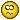#### Auri

##### 🎸🎼Metal Star🎼🎸
Safety & Support
SF Supporter
i now realize my assumption of y&z both=2 is incorrect
That is all I was disagreeing about, the rest was perfectly fine and understood.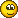------------------------------

I did mention that I assumed we only have integers as solutions (no fractions of apples on the plates) so the only solutions would be the ones I wrote above :

x = 4, y = 0, z = 4
x = 4, y = 1, z = 3
x = 4, y = 2, z = 2
x = 4, y = 3, z = 1
x = 4, y = 4, z = 0
Of course I didn't take negative amounts of apples either, lol, but I didn't mention that in my written text. These are not mathematical restrictions, just the interpretation of a real-life problem, right.

So more formally the solution is x = 4 and y + z = 4 for all positive integers (also called natural numbers), or what you said, which means the exact same thing (perfect!). All possibilities are listed above, there are 5 of them so I wrote them down. You can write this result in the form of inequalities indeed, that's up to your preferences.Like this?

x = 4​
0 ⩽ y ⩽ 4​
0 ⩽ z ⩽ 4​
for y, z ∈ ℕ​

or also :

x = 4​
y = [ 0 ; 4 ]​
z = [ 0 ; 4 ]​
for y, z ∈ ℕ​

(ℕ being natural numbers, or positive integers. Could have also used ℤ, which are all integers, as the inequalities already "say" that we only keep positive ones. This is detail that doesn't really matter anyway, it's just formal stuff. Sorry, I drank coffee, I may be overenthusiastic and sound like a jerky know-it-all, I never mean to... Pfff I hate myself. Never talk about maths or science with me. * facepalm *)

#### Dante

##### In the SF doghouse with Burt
SF Pro
SF Supporter
Still, lets see, so, your riddle states the following:

A is half of A + B + C, so Equation 1: X = Y + Z
Also, taking half of B and half of C from A gives you 2 apples, so Equation 2: X - (Y/2 + Z/2) = 2

Ok, time to figure it out.

First lets simplify Equation 2: Equation 2a: X - (Y+Z)/2 = 2
Then we can simply replace "B+C" in Eq 2a with the value of B+C shown in Eq 1, giving Equation 3: X - X/2 = 2
Equation 3 means X = 4 apples, meaning (From Eq 1) Y + Z = 4 apples.

The problem is that this is all the information you have, luckily A = 4 means there really arent that many permutations for what B and C could be, unfortunately with the current information all of the following work:

Y = 0 & Z = 4
Y = 1 & Z = 3
Y = 2 & Z = 2
Y = 3 & Z = 1
Y = 4 & Z = 0

Just sub these values into any of the equations derived from the question and it works, that alone proves there isnt enough information, but also, if you read the problem again you will see that there is no information comparing the values of Y and Z, no information comparing Y or Z to X individually, and indeed no information handling the values of Y and Z separately at all, (the 2nd part "If you take away 1/2 of the number of apples that lie on the second plate from the first plate, as well as 1/2 of the number of apples that lie on the third plate also from the first plate, then only 2 apples would be left over on the first plate." sounds like it is talking about them separately but "half of the second and half of the third" is the same as "half of the 2nd and 3rd together", thus treating them as 1 variable again, meaning there is no information about the individual values of Y and Z only the values of them together, meaning the above list is truly as far as you can get without more information or assumptions.

You solved for X and didnt get further because there isnt enough information to get further with any certainty, if this teenager DID, he did so with an assumption, which isnt maths, it is guesswork, I'm guessing the answer you have is X=4, Y=2, Z=2 right? without any info on the relation of Y to Z it is easy to just assume they are the same, but there is no proof of that. The fact that you couldn't get further proves you are better at maths than the 13 year old because you stopped when you ran out of information, you didn't just make up your own to get to the finish line.

#### Aurelia

##### I'm. Not. Like. You. I. Just. Fuck. Up.
Well you tell me the exact answer.Cause it seems like an indeterminate system (more than one solution) with too many unknowns given the information. We don't know anything about y and z.So if I understood correctly, it should get to all solutions verifying x = 4 and y + z = 4. Assuming we only have whole apples (so removing fractions, lol), it works for :

x = 4, y = 0, z = 4
x = 4, y = 1, z = 3
x = 4, y = 2, z = 2
x = 4, y = 3, z = 1
x = 4, y = 4, z = 0

I may be misunderstanding though, or there is a magic trick I'm missing, but that's what I have at first glance.

Yeah that kind of thing is taught around 13-14, but it doesn't make you dumb not to know how to solve it as an adult, we forget most of what we learn in school.
Motherfucker, O_O!!!! Why, that tricky little son of a bitch...(the kid's math teacher)...

The kid came up with 4, 2, and 2 for X, Y, and Z (respectively), and it made sense when I thought about it, so I didn't even consider the possibility that there could be more than one answer here. Un-freaking-believable. See, that's exactly what i came up with when i tried to do the problem. Same as you. That X has to be 4, the total number of apples has o be 8, and that Y + Z, therefore, has to equal 4. But where I got stuck was trying to figure out how Y and Z came out to equal 2, and I couldn't do it for the life of me. And now I can see why...because there's more than one freaking anwer!!! See, I knew you'd figure this shit out in a heartbeat, you smart-as-hell individual, you.

Status
Not open for further replies.

Total amount
\$0.00
Goal
\$255.00